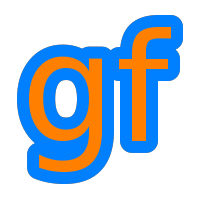Gamedev Framework (gf)  0.4.0 A C++11 framework for 2D games
gf::Penetration Struct Reference

Data about the collision between two objects. More...

#include <gf/Collision.h>

## Public Attributes

Vector2f normal
Collision normal. More...

float depth
Penetration depth. More...

## Related Functions

(Note that these are not member functions.)

bool collides (const CircF &lhs, const Transform &lhsTrans, const CircF &rhs, const Transform &rhsTrans, Penetration &p)
Check if two circles collides. More...

bool collides (const CircF &lhs, const CircF &rhs, Penetration &p)
Check if two circles collides. More...

bool collides (const RectF &lhs, const CircF &rhs, Penetration &p)
Check if a rectangle collides with a circle. More...

bool collides (const CircF &lhs, const RectF &rhs, Penetration &p)
Check if a circle collides with a rectangle. More...

bool collides (const RectF &lhs, const RectF &rhs, Penetration &p)
Check if two rectangles collides. More...

bool collides (const CircF &lhs, const Transform &lhsTrans, const Polygon &rhs, const Transform &rhsTrans, Penetration &p)
Check if a circle collides with a polygon. More...

bool collides (const CircF &lhs, const Polygon &rhs, Penetration &p)
Check if a circle collides with a polygon. More...

bool collides (const Polygon &lhs, const Transform &lhsTrans, const CircF &rhs, const Transform &rhsTrans, Penetration &p)
Check if a polygon collides with a circle. More...

bool collides (const Polygon &lhs, const CircF &rhs, Penetration &p)
Check if a polygon collides with a circle. More...

bool collides (const Polygon &lhs, const Transform &lhsTrans, const Polygon &rhs, const Transform &rhsTrans, Penetration &p)
Check if two polygons collides. More...

bool collides (const Polygon &lhs, const Polygon &rhs, Penetration &p)
Check if two polygons collides. More...

## Detailed Description

Data about the collision between two objects.

How to Create a Custom Physics Engine

## Friends And Related Function Documentation

 bool collides ( const CircF & lhs, const Transform & lhsTrans, const CircF & rhs, const Transform & rhsTrans, Penetration & p )
related

Check if two circles collides.

Parameters
 lhs First circle lhsTrans Transformation of the first circle rhs Second circle rhsTrans Transformation of the second circle p Data to fill if there is a collision
Returns
True if there is a collision
 bool collides ( const CircF & lhs, const CircF & rhs, Penetration & p )
related

Check if two circles collides.

Parameters
 lhs First circle rhs Second circle p Data to fill if there is a collision
Returns
True if there is a collision
 bool collides ( const RectF & lhs, const CircF & rhs, Penetration & p )
related

Check if a rectangle collides with a circle.

Parameters
 lhs The rectangle rhs The circle p Data to fill if there is a collision
Returns
True if there is a collision
 bool collides ( const CircF & lhs, const RectF & rhs, Penetration & p )
related

Check if a circle collides with a rectangle.

Parameters
 lhs The circle rhs The rectangle p Data to fill if there is a collision
Returns
True if there is a collision
 bool collides ( const RectF & lhs, const RectF & rhs, Penetration & p )
related

Check if two rectangles collides.

Parameters
 lhs First rectangle rhs Second rectangle p Data to fill if there is a collision
Returns
True if there is a collision
 bool collides ( const CircF & lhs, const Transform & lhsTrans, const Polygon & rhs, const Transform & rhsTrans, Penetration & p )
related

Check if a circle collides with a polygon.

Parameters
 lhs The circle lhsTrans Transformation of the circle rhs The polygon rhsTrans Transformation of the polygon p Data to fill if there is a collision
Returns
True if there is a collision
 bool collides ( const CircF & lhs, const Polygon & rhs, Penetration & p )
related

Check if a circle collides with a polygon.

Parameters
 lhs The circle rhs The polygon p Data to fill if there is a collision
Returns
True if there is a collision
 bool collides ( const Polygon & lhs, const Transform & lhsTrans, const CircF & rhs, const Transform & rhsTrans, Penetration & p )
related

Check if a polygon collides with a circle.

Parameters
 lhs The polygon lhsTrans Transformation of the polygon rhs The circle rhsTrans Transformation of the circle p Data to fill if there is a collision
Returns
True if there is a collision
 bool collides ( const Polygon & lhs, const CircF & rhs, Penetration & p )
related

Check if a polygon collides with a circle.

Parameters
 lhs The polygon rhs The circle p Data to fill if there is a collision
Returns
True if there is a collision
 bool collides ( const Polygon & lhs, const Transform & lhsTrans, const Polygon & rhs, const Transform & rhsTrans, Penetration & p )
related

Check if two polygons collides.

Parameters
 lhs First polygon lhsTrans Transformation of the first polygon rhs Second polygon rhsTrans Transformation of the second polygon p Data to fill if there is a collision
Returns
True if there is a collision
 bool collides ( const Polygon & lhs, const Polygon & rhs, Penetration & p )
related

Check if two polygons collides.

Parameters
 lhs First polygon rhs Second polygon p Data to fill if there is a collision
Returns
True if there is a collision

## Member Data Documentation

 float gf::Penetration::depth

Penetration depth.

 Vector2f gf::Penetration::normal

Collision normal.